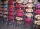# Hussein

Hussein owns 450000. He spent at the bookstore 2 over 9 to buy some books and tales. He paid 3 over 5 of his money to buy his math book. Then he paid his money to buy stories diagram ;
a. Calculate the remaining amount of money with Hussein?
b. Hussein lost 3 over 4 of the remaining money. Calculate the amount of money lost?

Result

a =  80000
b =  60000

#### Solution:Leave us a comment of example and its solution (i.e. if it is still somewhat unclear...):

Showing 1 comment:Math student
Your English is not good,.#### To solve this example are needed these knowledge from mathematics:

Need help calculate sum, simplify or multiply fractions? Try our fraction calculator.

## Next similar examples:

1. Cris hadCris had 15000 . He spent 1/3 of his money on a table and the remaining on 5 similar chairs that cost the same. How much did one chair cost?
2. WithdrawalIf I withdrew 2/5 of my total savings and spent 7/10 of that amount. What fraction do I have in left in my savings?
3. ZdeněkZdeněk picked up 15 l of water from a 100-liter full-water barrel. Write a fraction of what part of Zdeněk's water he picked.
4. Fraction to decimalWrite the fraction 3/22 as a decimal.
5. Mixed2improperWrite the mixed number as an improper fraction. 166 2/3
6. Fraction and a decimalWrite as a fraction and a decimal. One and two plus three and five hundredths
7. Lengths of the poolMiguel swam 6 lengths of the pool. Mat swam 3 times as far as Miguel. Lionel swam 1/3 as far as Miguel. How many lengths did mat swim?
8. In fractionsAn ant climbs 2/5 of the pole on the first hour and climbs 1/4 of the pole on the next hour. What part of the pole does the ant climb in two hours?
9. PercentagesWhat is 1/3 of \$821.43?
10. Product of two fractionsProduct of two fractions is 9 3/5 . If one of the fraction is 9 3/7. Find the other fraction.
11. One-third 2One-third of the people in a barangay petitioned the council to allow them to plant in vacant lots and another 1/5 of the people petitioned to have a regular garbage collection. What FRACTION of the barangay population made the petition?
12. Ratio 11Simplify this ratio 10 : 1/4
13. Pizza 5You have 2/4 of a pizza and you want to share it equally between 2 people how much pizza does each person get?
14. CakesOn the bowl were a few cakes. Jane ate one-third of them, Dana ate a quarter of those cakes that remained. a) What part (of the original number of cakes) Dana ate? b) At least how many cakes could be (initially) on thebowl?
15. Fractions 3Calculate 1/9 of 27:
16. One frame5 picture frames cost € 12 more than three frames. How much cost one frame?
17. Water 31Richard takes 3 1/6 liters of water before noon and 2 3/5 liters of water after noon. How many litres of water does Richard consume a day ?